Data Handling (Mathematics) Class 6 - NCERT Questions
Q 1.

In a Mathematics test, the following marks were obtained by 40 students. Arrange these marks in a table using tally marks.
 8 1 3 7 6 5 5 4 4 2 4 9 5 3 7 1 6 5 2 7 7 3 8 4 2 8 9 5 8 6 7 4 5 6 9 6 4 4 6 6

(A) Find how many students obtained marks equal to or more than 7.
(B) How many students obtained marks below 4?

SOLUTION: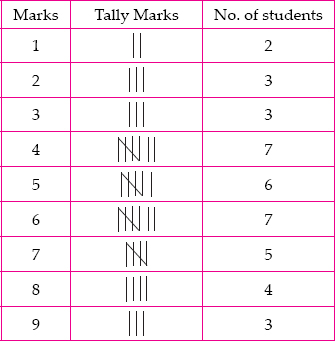(A) 5 + 4 + 3 = 12 students obtained marks equal to or more than 7.
(B) 2 + 3 + 3 = 8 students obtained marks
below 4.

Q 2.

Follo09wing is the choice of sweets of 30 students of Class VI.
(A) Arrange the names of sweets in a table using tally marks.
(B) Which sweet is preferred by most of the students?

SOLUTION: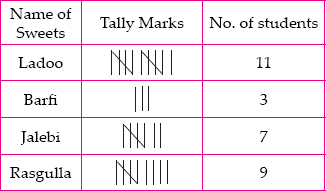(B) Ladoo is preferred by most of the students.

Q 3.

Catherine threw a dice 40 times and noted the number appearing each time as shown below:
 1 3 5 6 6 3 5 4 1 2 5 3 4 6 1 5 5 6 1 1 2 2 3 5 2 4 5 5 6 5 1 6 2 3 5 2 4 1 5

Make a table and enter the data using tally marks. Find the number that appeared
(A) The minimum number of times.
(B) The maximum number of times.
(C) Find those numbers that appear an equal number of times.

SOLUTION: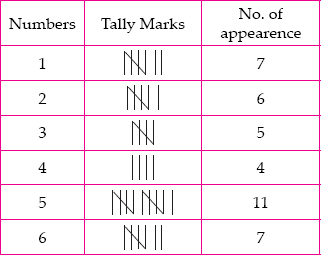(A) 4 appeared minimum number of times.
(B) 5 appeared maximum number of times.
(C) 1 and 6 appeared equal number of times.

Q 4.

Following pictograph shows the number
of tractors in five villages.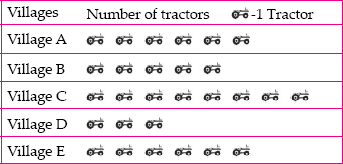Observe the pictograph and answer the following questions.
(i) Which village has the minimum number of tractors?
(ii) Which village has the maximum number of tractors?
(iii) How many more tractors village C has as compared to village B.
(iv) What is the total number of tractors in all the five villages?

SOLUTION:

(i) Village D has the minimum number of tractors.
(ii) Village C has the maximum number of tractors.
(iii) Village C has 8 – 5 = 3 more tractors than village B.
(iv) Total number of tractors = 6 + 5 + 8 + 3 + 6 = 28

Q 5.

The number of girl students in each class of a co-educational middle school is depicted by the pictograph :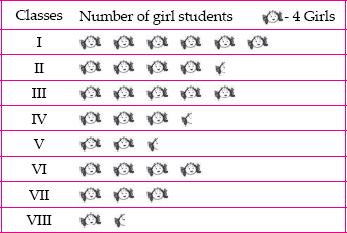Observe this pictograph and answer the following questions :
(A) Which class has the minimum number of girl students?
(B) Is the number of girls in Class VI less than the number of girls in Class V?
(C) How many girls are there in Class VII?

SOLUTION:

 Classes Number of girl students I 6 × 4 = 24 II 4 × 4 + 2 = 18 III 5 × 4 = 20 IV 3 × 4 + 2 = 14 V 2 × 4 + 2 = 10 VI 4 × 4 = 16 VII 3 × 4 = 12 VIII 1 × 4 + 2 = 6 Total 120

(A) Class VIII has the minimum number of girl students.
(B) No, the number of girls in class VI is greater than the number of girls in class V.
(C) There are 12 girls in class VII.

Q 6.

The sale of electric bulbs on different days of a week is shown below :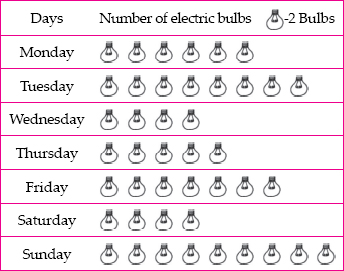Observe the pictograph and answer the following questions :
(A) How many bulbs were sold on Friday?
(B) On which day were the maximum number of bulbs sold?
(C) On which of the days same number of bulbs were sold?
(D) On which of the days minimum number of bulbs were sold?
(E) If one big carton can hold 9 bulbs. How many cartons were needed in the given week?

SOLUTION:

 Days Number of bulbs Monday 6 × 2 = 12 Tuesday 8 × 2 = 16 Wednesday 4 × 2 = 8 Thursday 5 × 2 = 10 Friday 7 × 2 = 14 Saturday 4 × 2 = 8 Sunday 9 × 2 = 18 Total 86

(A) Number of bulbs sold on Friday is 14.
(B) Maximum number of bulbs were sold on Sunday.
(C) Same number of bulbs were sold on Wednesday and Saturday.
(D) Minimum number of bulbs were sold on Wednesday and Saturday.
(E) The total number of bulbs were sold in the given week = 86
Number of cartons required for 9 bulbs = 1
∴ Number of cartons required for 86 bulbs
= 86 ÷ 9 = 9.5510
Therefore, 10 cartons were needed in the given week.

Q 7.

In a village six fruit merchants sold the following number of fruit baskets in a particular season: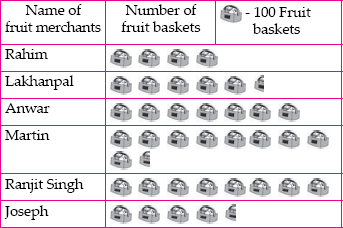Observe this pictograph and answer the following questions :
(A) Which merchant sold the maximum number of baskets?
(B) How many fruit baskets were sold by Anwar?
(C) The merchants who have sold 600 or more number of baskets are planning to buy a godown for the next season. Can you name them?

SOLUTION:

 Name of fruit merchants Number of fruit baskets Rahim 4 × 100 = 400 Lakhanpal 5 × 100 + 50 = 550 Anwar 7 × 100 = 700 Martin 9 × 100 + 50 = 950 Ranjit Singh 8 × 100 = 800 Joseph 4 × 100 + 50 = 450 Total 3850

(A) Martin sold the maximum number of baskets.
(B) 700 fruit baskets were sold by Anwar.
(C) Anwar, Martin and Ranjit Singh have sold more than 600 baskets.

Q 8.

Total number of animals in five villages are as follows :
 Village A : 80 Village C : 90 Village E : 60 Village B : 120 Village D : 40

Prepare a pictograph of these animals using one symbol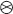to represent 10 animals and answer the following questions :
(A) How many symbols represent animals of village E?
(B) Which village has the maximum number of animals?
(C) Which village has more animals : village A or village C?

SOLUTION: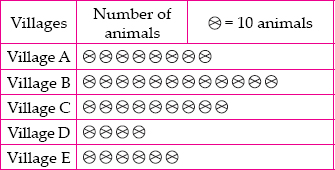(A) 6 symbols represent animals of village E.
(B) Village B has the maximum number of animals.
(C) Village C has more animals than village A.

Q 9.

Total number of students of a school in different years is shown in the following table.
 Years Number of students 1996 400 1998 535 2000 472 2002 600 2004 623

A. Prepare a pictograph of students using one symbol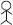to represent 100 students and answer the following questions:
(A) How many symbols represent total number of students in the year 2002?
(B) How many symbols represent total number of students for the year 1998?
B. Prepare another pictograph of students using any other symbol each representing 50 students. Which pictograph do you find more informative?

SOLUTION:

A.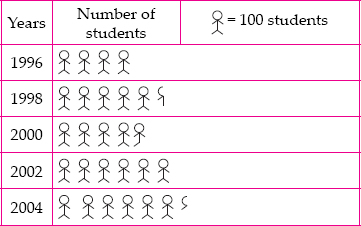(A) 6 symbols represent total number of students in the year 2002.
(B) Five completed and one incomplete symbols represent total number of students for the year 1998.
B.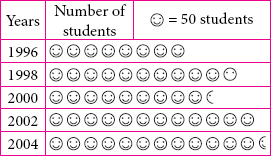Q 10.

The given bar graph shows the amount of
wheat purchased by government during the year 1998-2002.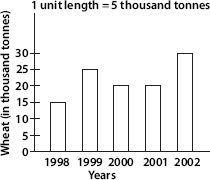Read the bar graph and write down your observations. In which year was
(A) the wheat production maximum?
(B) the wheat production minimum?

SOLUTION:

(A) In 2002, production of wheat was maximum.
(B) In 1998, production of wheat was minimum.

Q 11.

Observe this bar graph which is showing the sale of shirts in a ready made shop from Monday to Saturday.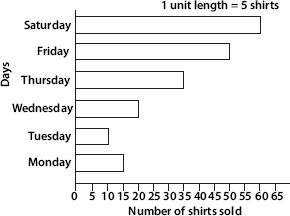Now answer the following questions :
(A) What information does the above bar graph give?
(B) What is the scale chosen on the horizontal line representing number of shirts?
(C) On which day were the maximum number of shirts sold? How many shirts were sold on that day?
(D) On which day were the minimum number of shirts sold?
(E) How many shirts were sold on Thursday?

SOLUTION:

(A) The bar graph shows the sale of shirts in a ready made shop from Monday to Saturday.
(B) 1 unit length = 5 shirts
(C) On Saturday, maximum number of shirts, i.e., 60 shirts were sold.
(D) On Tuesday, minimum number of shirts were sold.
(E) On Thursday, 35 shirts were sold.

Q 12.

Observe this bar graph which shows the marks obtained by Aziz in half-yearly examination in different subjects.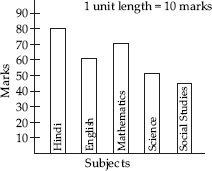(A) What information does the bar graph give?
(B) Name the subject in which Aziz scored maximum marks.
(C) Name the subject in which he has scored minimum marks.
(D) State the name of the subjects and marks obtained in each of them.

SOLUTION:

(A) The bar graph shows the marks obtained by Aziz in half-yearly examination in different subjects.
(B) Hindi
(C) Social Studies
(D) Hindi-80, English-60, Mathematics-70, Science-50, Social Studies-40.

Q 13.

A survey of 120 school students was done to find which activity they prefer to do in their free time.
 Preferred activity Number of students Playing 45 Reading story books 30 Watching TV 20 Listening to music 10 Painting 15

Draw a bar graph to illustrate the above data taking scale of 1 unit length = 5 students.
Which activity is preferred by most of the students other than playing?

SOLUTION: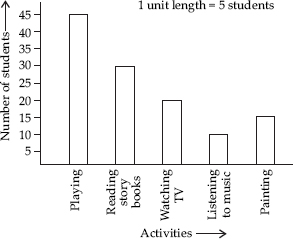Reading story books is preferred by most of the students other than playing.

Q 14.

The number of Mathematics books sold by a shopkeeper on six consecutive days is shown below :
 Days Sunday Monday Tuesday Wednesday Thursday Friday Number of books sold 65 40 30 50 20 70
Draw a bar graph to represent the above information choosing the scale of your choice.

SOLUTION: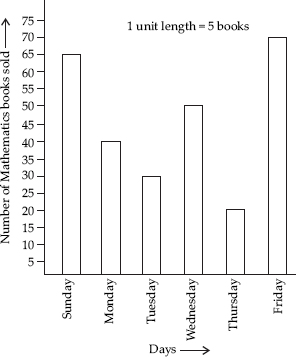Q 15.

Following table shows the number of bicycles manufactured in a factory during the years 1998 to 2002. Illustrate this data using a bar graph. Choose a scale of your choice.
 Years Number of bicycles manufactured 1998 800 1999 600 2000 900 2001 1100 2002 1200

(A) In which year were the maximum number of bicycles manufactured?
(B) In which year were the minimum number of bicycles manufactured?

SOLUTION: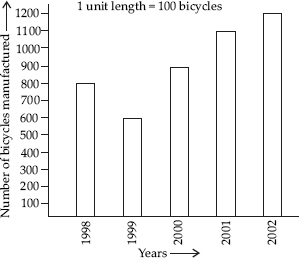(A) In 2002, the maximum number of bicycles were manufactured.
(B) In 1999, the minimum number of bicycles were manufactured.

Q 16.

Number of persons in various age groups in a town is given in the following table.
 Age group 1-14 15-29 30-44 45-59 60-74 75 and above Number of persons 2 lakhs 1 lakh 60 thousands 1 lakh 20 thousands 1 lakh 20 thousands 80 thousands 40 thousands

Draw a bar graph to represent the above information and answer the following questions. (take 1 unit length = 20 thousands)
(A) Which two age groups have same population?
(B) All persons in the age group of 60 and above are called senior citizens. How many senior citizens are there in the town?

SOLUTION: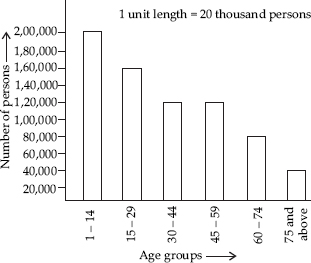(A) Age groups 30 – 44 and 45 – 59 have same population.
(B) 80,000 + 40,000 = 1,20,000 senior citizens are there in the town.